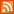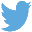Bucaro TecHelpHTTPS Encryption not required because no account numbers orpersonal information is ever requested or accepted by this siteCustom Search# SVG Example Code to Rotate Elements

```<svg width="340" height="350" version="1.1" xmlns="http://www.w3.org/2000/svg" style="border-style:solid;">
<rect x="0" y="0" width="100" height="100" stroke="#000" fill="red" />
<rect x="0" y="0" width="100" height="100" stroke="#000" fill="red" transform="rotate(45)" />

<rect x="200" y="200" width="100" height="100" stroke="#000" fill="green" />
<rect x="200" y="200" width="100" height="100" stroke="#000" fill="green" transform="rotate(45 200 200)" />

<rect x="200" y="0" width="50" height="50" stroke="#000" fill="yellow" />
<rect x="200" y="0" width="50" height="50" stroke="#000" fill="yellow" transform="rotate(45)" />
</svg>
```

The rotate transform rotates an element about a given coordinate by a specified angle. The syntax of the rotate transform is shown below.

transform="rotate(a x y)"

rotate takes three parameters. The first parameter specifies the degrees of rotation about a given point. The second and third parameters specify the coordinate point to rotate the element about. If the second and third parameters are not supplied, the rotate is about the origin.

In the above example, the upper-right corner of the first red square is located at the origin x=0 y=0, the upper-right corner of the second red square is also located at the origin, and then transform="rotate(45)" is applied (rotate 45 degrees around the origin), so the second red square is rotated around its upper-right corner around the orign by 45 degrees.

The upper-right corner of the first green square is located at coordinates x=200 y=200, the upper-right corner of the second green square is also located at x=200 y=200, and then transform="rotate(45 200 200)" is applied (rotate 45 around degrees coordinates x=200 y=200), so the second green square is rotated around its upper-right corner around the coordinates x=200 y=200 by 45 degrees.

The upper-right corner of the first yellow square is located at coordinates x=200 y=0, the upper-right corner of the second yellow square is also located at x=200 y=0, and then transform="rotate(45)" is applied (rotate 45 around degrees around the origin), so the upper-right corner of the second yellow square is rotated around the origin by 45 degrees.

 .menubtn { margin-top:10px; margin-left:50px; width:220px; opacity:1; border-style:solid; border-color:#009000; background-color:#66ff66; transition-property: opacity; transition-duration: 1s; transition-timing-function: ease-out; } .menubtn:hover { opacity:1; background-color:#e5ff23; } Menu - More Web Design GraphicsRSS FeedFollow @Stephen Bucaro[Site User Agreement] [Privacy Policy] [Site map] [Search This Site] [Contact Form]
Copyright©2001-2020 Bucaro TecHelp 13771 N Fountain Hills Blvd Suite 114-248 Fountain Hills, AZ 85268